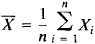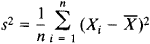Consistent Estimate

consistent estimate

[kən′sis·tənt ′es·tə·mət]
(statistics)
A method of estimation which has the property that the estimate is practically certain to fall very close to a parameter being estimated, provided there are sufficient observations.

Consistent Estimate

a type of statistical estimate of a parameter of a probability distribution. A consistent estimate has the property that as the number of observations increases, the probability of the estimate deviating from the estimated parameter by more than some assigned number approaches 0. For a more precise definition, let X1X2, Xn be independent observation results whose distribution is a function of an unknown parameter θ, and, for every n, let the function Tn = Tn(X1, . . . , Xn) be an estimate of θ constructed from the first n observations. The sequence of estimates (Tn) is then said to be consistent if, for every arbitrary number ∊ > 0 and for any permissible value of θ,

P{ǀTn – θǀ > ∊}→ 0

as n → ∞. In other words, the estimate is consistent if Tn converges in probability to θ.

Any unbiased estimate Tn of θ (or estimate with ETn → 0) whose variance approaches 0 with increasing n is a consistent estimate of θ by virtue of Chebyshev’s inequality

PTn – θǀ>∊)<DTn/∊2

Thus, the sample meanand the sample varianceare consistent estimates of the mathematical expectation and variance, respectively, of a normal distribution.

Although a desirable characteristic of every statistical estimate, consistency pertains only to the asymptotic properties of the estimate. In practical applications with finite sample sizes, the consistency of an estimate does not necessarily mean the estimate is a good one. Criteria exist for selecting from various consistent estimates of some parameter the estimate that exhibits the desired properties.

The concept of a consistent estimate was first proposed by the British mathematician R. Fisher in 1922.

REFERENCES

Cramer, H. Matematicheskie melody statistiki. Moscow, 1975. (Translated from English.)
Rao, C. R. Lineinye statisticheskie melody i ikh primeneniia. Moscow, 1968. (Translated from English.)

A. V. PROKHOROV

References in periodicals archive ?
The utility of reconstructing the unobserved component of the historical ocean state is patent, and so-called reanalyses of the ocean and atmosphere that provide a gridded, gap-free and dynamically consistent estimate of the historical system state, have found extremely broad use across the sciences (e.
In Figure 1a, control for C (0) through regression, stratification, or restriction provides a consistent estimate of the C (0)--conditional causal effect of exposure A (0) on outcome B (2).
A consistent estimate of [tau] has been obtained in accordance with the procedure explicated by Chan (1993).
Lead author Alessandro Baccini, an assistant scientist at WHRC, explained that the new data set provides a spatially and temporally consistent estimate of carbon stock and a stronger foundation for estimating carbon emissions by better characterizing the carbon density of the forest that has been lost.
Input-output identification in the field of linear least squares methods is, as far as consistent estimate is concerned, sufficiently developed.
In the case of the TVC procedure followed in this article, however, we then decompose these varying coefficients into two parts: a consistent estimate of the true structural partial derivative and the remaining part that is due to biases from the various misspecifications in the model.
Last year's July/August "Japan Insider" column highlighted the state of the herbs and botanicals market in Japan and how difficult it is to obtain an accurate and consistent estimate of the size of the Japanese retail market for herbs and botanicals.
It is now possible to obtain a consistent estimate if we apply the generalised least squares to equation (5).
This suggests that regression methods, which use the observed covariation between worker productivity and the payment system to identify the incentive effect, may fail to provide a consistent estimate of this effect.
B] (of either [Beta] or [Beta][prime]) provides a consistent estimate of how the minimum wage effect changes over time.
jms] is a consistent estimate of [Alpha][s, j (m)] - [Alpha][+, j (m)]where [Alpha][+, j (m)] is the average intrinsic preference for brand j across all segments.
Since the OLS estimates of expression 1 are consistent, we can use the OLS residuals from that estimation to provide a consistent estimate of [sigma].

Site: Follow: Share:
Open / Close